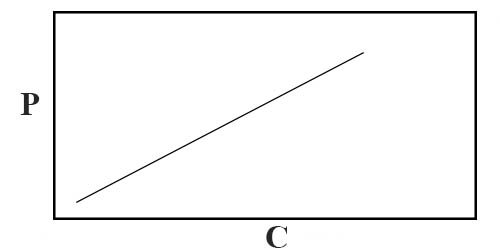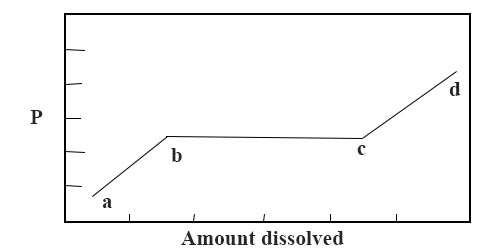Chemistry

# Solution of Gas in SolidSolution of Gas in Solid

Gases are always taken up by solids but the extent of uptake varies widely. It the uptake is by adsorption only the uptake is quite small and this phenomenon in general cannot be taken as solution. There are three other ways in which the gases can be taken up by solids:

(i) A gas may be distributed uniformly giving rise to a homogeneous solution in such a way that there is no change in the molecular structure or composition of the gas. Such cases constitute true solutions and they resemble the solution of gas in liquid closely. Solutions of ammonia and sulphur dioxide on charcoal are examples of such cases. There is a decrease in the translational Kinetic energy of the molecules while they dissolve in the solid and such solutions are exothermic in nature. These systems obey Henry’s law like the solutions of gas in liquid. If P be the pressure at which the gas is dissolved at a constant temperature then the concentration of the gas in the solution is directly proportional to the pressure. If the concentration of the gas in the solid is C, then P/C = constant and a plot of P vs C will be a straight line, as shown in Figure 1. If the gas undergoes as sociation in the solution and if n is the degree of association, then, P/n√C = constant, whereas in case of dissociation the relation becomes, P/Cn = constant, where n now denotes the number of parts into which the molecule is dissociated.Figure: P vs C for solution of gas in solid

(ii) If the gas forms a solid solution a different behavior is observed as shown in Figure 2.The part ab represents the usual true solution and the relation P/C = K holds until the point b is reached. At b and up to c, a new solid solution is formed. Since the curve is an isothermal the system is invariant. Therefore, bc runs parallel to the pressure axis. On increasing the pressure still, further, this solid solution disappears at t and the usual relation P/C = K is followed along cd except that the straight line does not pass through the origin.Fig: P vs amount of gas dissolved in solid

(iii) The third type of uptake of a gas by a solid is due to the compound formation and no general conclusions are possible unless the dissociation pressure of the solid compound so formed is considered. Thus uptake of carbon dioxide by calcium oxide is an example of compound formation giving calcium carbonate.